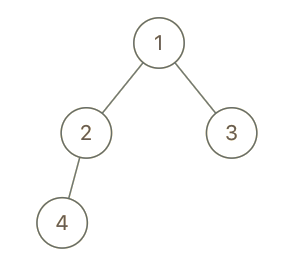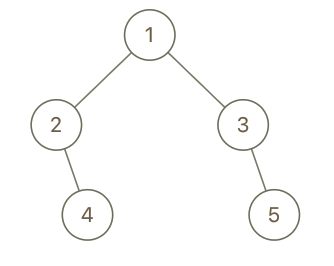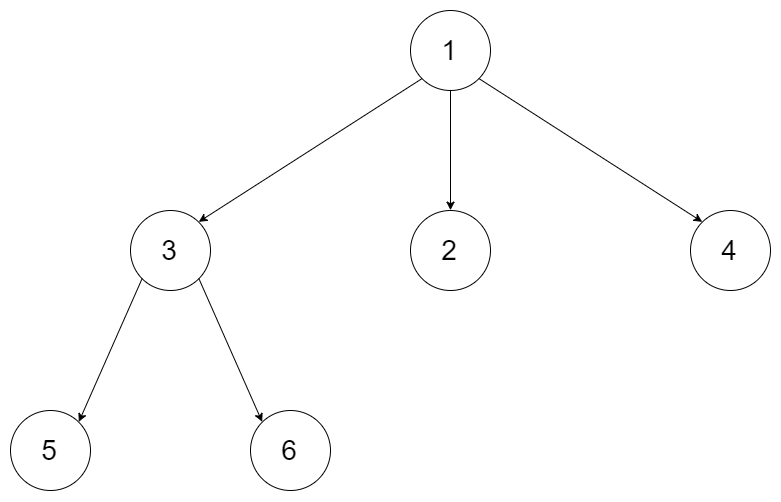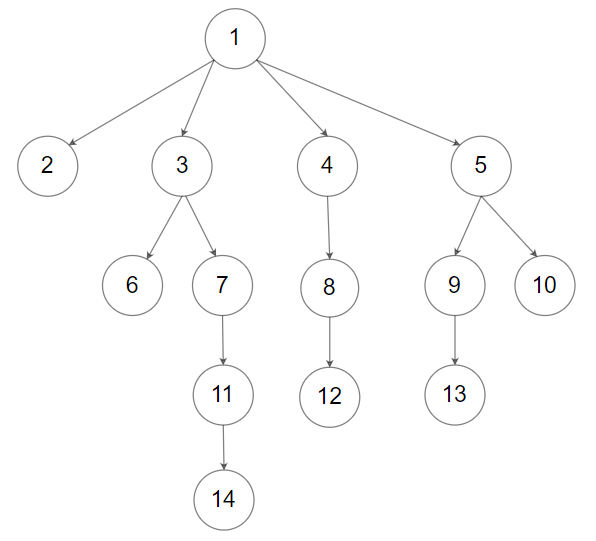# leetcode 200. Number of Islands

## 题目描述

Given a 2d grid map of `'1'`s (land) and `'0'`s (water), count the number of
islands. An island is surrounded by water and is formed by connecting adjacent
lands horizontally or vertically. You may assume all four edges of the grid
are all surrounded by water.

Example 1:

``````Input:
11110
11010
11000
00000

Output:  1``````

Example 2:

``````Input:
11000
11000
00100
00011

Output: 3``````

Tags: Depth-first Search, Breadth-first Search, Union Find

Difficulty: Medium



# leetcode 993. Cousins in Binary Tree

## 题目描述

In a binary tree, the root node is at depth `0`, and children of each depth
`k` node are at depth `k+1`.

Two nodes of a binary tree are cousins if they have the same depth, but have
different parents.

We are given the `root` of a binary tree with unique values, and the values
`x` and `y` of two different nodes in the tree.

Return `true` if and only if the nodes corresponding to the values `x` and `y`
are cousins.

Example 1:``````Input: root = [1,2,3,4], x = 4, y = 3
Output: false``````

Example 2:``````Input: root = [1,2,3,null,4,null,5], x = 5, y = 4
Output: true``````

Example 3:``````Input: root = [1,2,3,null,4], x = 2, y = 3
Output: false``````

Note:

1. The number of nodes in the tree will be between `2` and `100`.
2. Each node has a unique integer value from `1` to `100`.

Tags: Tree, Breadth-first Search

Difficulty: Easy

# leetcode 559. Maximum Depth of N-ary Tree

## 题目描述

Given a n-ary tree, find its maximum depth.

The maximum depth is the number of nodes along the longest path from the root
node down to the farthest leaf node.

Nary-Tree input serialization is represented in their level order traversal,
each group of children is separated by the null value (See examples).

Example 1:Input: root = [1,null,3,2,4,null,5,6] Output: 3

Example 2:Input: root = [1,null,2,3,4,5,null,null,6,7,null,8,null,9,10,null,null,11,null,12,null,13,null,null,14] Output: 5

Constraints:

• The depth of the n-ary tree is less than or equal to `1000`.
• The total number of nodes is between `[0, 10^4]`.

Tags: Tree, Depth-first Search, Breadth-first Search

Difficulty: Easy

# leetcode 279. Perfect Squares

## 题目描述

Given a positive integer n , find the least number of perfect square numbers
(for example, `1, 4, 9, 16, ...`) which sum to n.

Example 1:

``````Input: _n_ = 12
Output: 3
Explanation:12 = 4 + 4 + 4.``````

Example 2:

``````Input: _n_ = 13
Output: 2
Explanation:13 = 4 + 9.``````

Tags: Math, Dynamic Programming, Breadth-first Search

Difficulty: Medium

# leetcode 111. Minimum Depth of Binary Tree

## 题目描述

Given a binary tree, find its minimum depth.

The minimum depth is the number of nodes along the shortest path from the root
node down to the nearest leaf node.

Note: A leaf is a node with no children.

Example:

Given binary tree `[3,9,20,null,null,15,7]`,

``````  3
/ \
9  20
/  \
15   7``````

return its minimum depth = 2.

Tags: Tree, Depth-first Search, Breadth-first Search

Difficulty: Easy

# leetcode 107. Binary Tree Level Order Traversal II

## 题目描述

Given a binary tree, return the bottom-up level order traversal of its
nodes’ values. (ie, from left to right, level by level from leaf to root).

For example:
Given binary tree `[3,9,20,null,null,15,7]`,

``````  3
/ \
9  20
/  \
15   7``````

return its bottom-up level order traversal as:

``````[
[15,7],
[9,20],

]``````

Tags: Tree, Breadth-first Search

Difficulty: Easy

# leetcode 103. Binary Tree Zigzag Level Order Traversal

## 题目描述

Given a binary tree, return the zigzag level order traversal of its nodes’
values. (ie, from left to right, then right to left for the next level and
alternate between).

For example:
Given binary tree `[3,9,20,null,null,15,7]`,

``````  3
/ \
9  20
/  \
15   7``````

return its zigzag level order traversal as:

``````[
,
[20,9],
[15,7]
]``````

Tags: Stack, Tree, Breadth-first Search

Difficulty: Medium

:D 一言句子获取中...